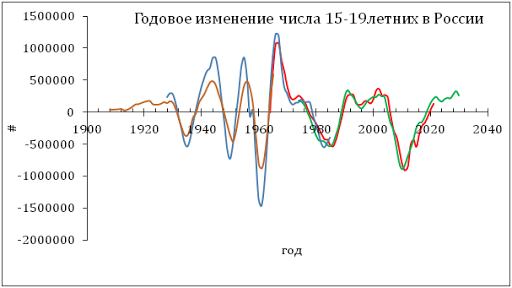## 1/26/12

### Why the Economic Projections of Federal Reserve Board are inconsistent

The FRB members have recently projected the evolution of key macroeconomic variables including real GDP and the rate of unemployment. In our blog , we have developed a very accurate model linking the rate of unemployment in the US to the rate of real GDP (per capita) growth: (A series of posts has resulted in a working paper.) The following relationship was estimated:

du = -0.62dlnG + 1.09,  (1)

When integrated between t0 and t, equation (1) can be rewritten in the following form:

u(t) = u(t0) + bln[G/G0] +a(t-t0) + c  (2)

Without loss of generality, we assume t0=0. The intercept c≡0, as is clear for t=t0. Instead of integrating (2), we calculate cumulative sums of the annual estimates of du and lnG with appropriate initial conditions. The cumulative sum of du’s is the time series of the unemployment rate. Figure 1 depicts the measured and observed curves for the period between 1958 and 2011. The agreement is excellent and has been obtained by a formal statistical method (LSQR).

From (1) it follows that higher rates of GDP growth decrease the rate of unemployment. The FRB has projected real GDP with the highest rates of 2.7% in 2012, 3.2% in 2013, and 4% in 2014. We reduce these rates by 1% per year to estimate the per capita rate of growth, i.e. the growth in population is 1% per year. Using (2) we calculate the rate of uneployment which will correspond to the projected real GDP.
Figure 1 also depicts these predicted rates for 2012 to 2014 by open circles. The rates of unemployment projected by the FRB are shown by red circles. There is a significant deviation between the predicted and projected rates, which likely manifests the inconsistency in the FRB member's models of unemployment.

One may check these projections in 2015.

Figure 1. The observed and predicted rate of unemployment in the USA between 1958 and 2010.The projected rate of unemployment (middle point of the projections) is shown by red circles.